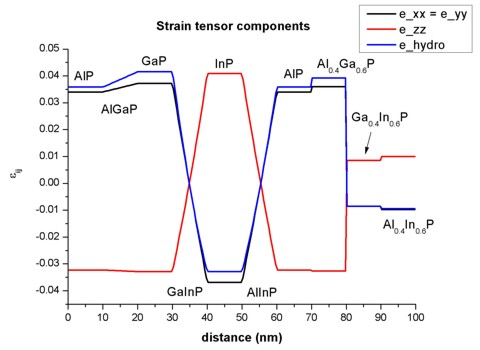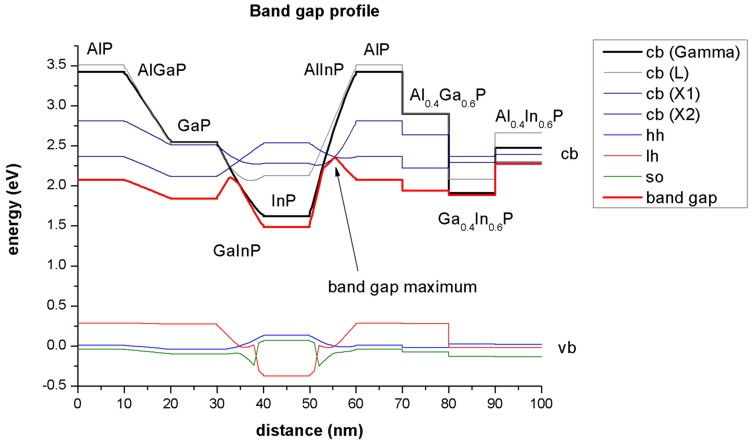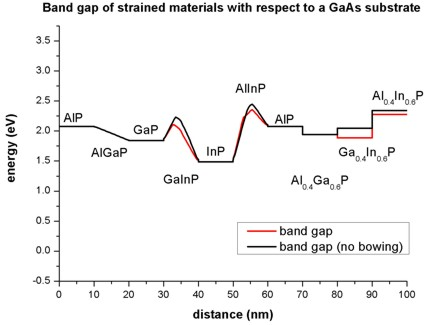nextnano.com  nextnano³  Download | Search | Copyright | Publications  * password protected nextnano³ software1D AlGaInP on GaAs

# nextnano3 - Tutorial

## Band gap of strained AlGaInP on GaAs substrate

```-> AlGaInP_on_GaAs_1D_as_in_tutorial_nn3.in -> AlGaInP_on_GaAs_1D_as_in_tutorial_no_bowing_nn3.in -> AlGaInP_on_GaAs_1D_nn3.in / *_nnp.in  - ```input file for the nextnano3 and nextnano++ software``` -> AlGaInP_on_GaAs_1D.xls```

## Band gap of strained AlGaInP on GaAs substrate

In this tutorial we want to study the band gaps of strained AlxGayIn1-x-yP on a GaAs substrate.

The material parameters are taken from
Band parameters for III-V compound semiconductors and their alloys
I. Vurgaftman, J.R. Meyer, L.R. Ram-Mohan
J. Appl. Phys. 89 (11), 5815 (2001)

To understand the effect of strain on the band gap on the individual components of this quaternary, we first examine the effects on
` 1) `AlP            strained tensilely          with respect to GaAs
` 2) `GaP           strained tensilely          with respect to GaAs
` 3) `InP             strained compressively with respect to GaAs
` 4) `AlxGa1-xP   strained tensilely          with respect to GaAs
` 5) `GaxIn1-xP    strained                       with respect to GaAs
` 6) `AlxIn1-xP     strained                       with respect to GaAs
` 7) `Al0.4Ga0.6P strained tensilely         with respect to GaAs
` 8) `Ga0.4In0.6P strained compressively with respect to GaAs
` 9) `Al0.4In0.6P  strained compressively with respect to GaAs

Each material layer has a length of 10 nm in the simulation.

The material layers 4), 5) and 6) vary its alloy contents linearly:
` 4) `AlxGa1-xP   from 10 nm to 20 nm from x=0.0 to x=1.0
` 5) `GaxIn1-xP    from 30 nm to 40 nm from x=0.0 to x=1.0
` 6) `AlxIn1-xP     from 50 nm to 60 nm from x=1.0 to x=0.0

### Strain

`-> AlGaInP_on_GaAs_1D_as_in_tutorial_nn3.in`

There is no external stress applied to the structure, so Poisson's ratio holds.
All layers are strained pseudomorphically with respect to a GaAs substrate (i.e. the layers are biaxially strained in the plane perpendicular to the growth direction to match the lattice constant of GaAs).

The biaxial strain in the layers can be calculated with this formula:
exx = eyy = (asubstrate - a) / a
where a is the lattice constant.

The output of the strain tensor can be found in this file:
`=> strain1/strain_cr1D.dat`

The hydrostatic strain is the trace of the strain tensor and corresponds to the volume deformation:
ehydro = Tr(eij) = exx + eyy + ezz### Band gaps

The following figure shows the conduction band edges at the Gamma, L and X points and the heavy hole, light hole and split-off hole valence bands.
The red line shows that band gap, i.e. the difference between the lowest conduction band minimum and the valence band maximum.
The band gap maximum occurs at Al0.55In0.45P (2.355 eV).

The conduction and valence band edges have been obtained taking into account the shifts and splittings of the bands due to strain and deformation potentials.

Note that conduction and valence band offsets are not taken into account in this plot.
The zero of energy was taken to be the unstrained heavy hole / light hole band edge.

Due to strain, the degeneracy of the heavy and light hole is lifted. Also, the X band splits into two X bands (2 fold and 4 fold degeneracy).

In the case of tensile (compressive) strain, the light (heavy) hole band is the valence band maximum.Note that the material parameters include band gap bowing.

`-> AlGaInP_on_GaAs_1D_as_in_tutorial_nn3_no_bowing.in`

The following figure compares the overall band gap to the case where band gap bowing has been neglected.• The nextnano3 software does not include quaternaries.
However, there is the option to simulate lattice-matched quaternaries.
They only have one variable x (and not two, x and y, as for a general quaternary) and are thus represented as "ternaries" with only one variable x.

```!---------------------------------------------------------------------------! ! AlGaInP lattice matched to GaAs --- Source: Vurgaftman et al., J. Appl. Phys. 89 (11), 5815 (2001) ! (Al(x)Ga(1-x))0.51In0.49P ! (Al0.52In0.48P)(x)(Ga0.51In0.49P)(1-x) !---------------------------------------------------------------------------!  material-name = (Al(x)Ga(1-x))0.51In0.49P  ```
• The nextnano++ software supports quaternaries.
``` quaternary_constant{     name = "Al(x)Ga(y)In(1-x-y)P"     alloy_x = 0.255     alloy_y = 0.255  } ```So if you are interested in quaternaries, this software should be preferred.
• The input files` *_as_in_tutorial_*.in `match the results from the above documented tutorial.
Note that in the tutorial, conduction and valence band offsets are not taken into account.
The zero of energy was taken to be the unstrained heavy hole / light hole band edge for all materials.
The nextnano++ input file (`*_nnp.in`) includes a quaternary material (between 100 nm and 110 nm).
The corresponding nextnano3 input file (`*_nn3.in`) includes a lattice matched quaternary material (between 100 nm and 110 nm).
Note that in these input files, conduction and valence band offsets are taken into account - in contrast to the figures in the tutorial.
• Appendix E of the PhD thesis of T. Zibold is related to the nextnano++ implementation of quaternaries.